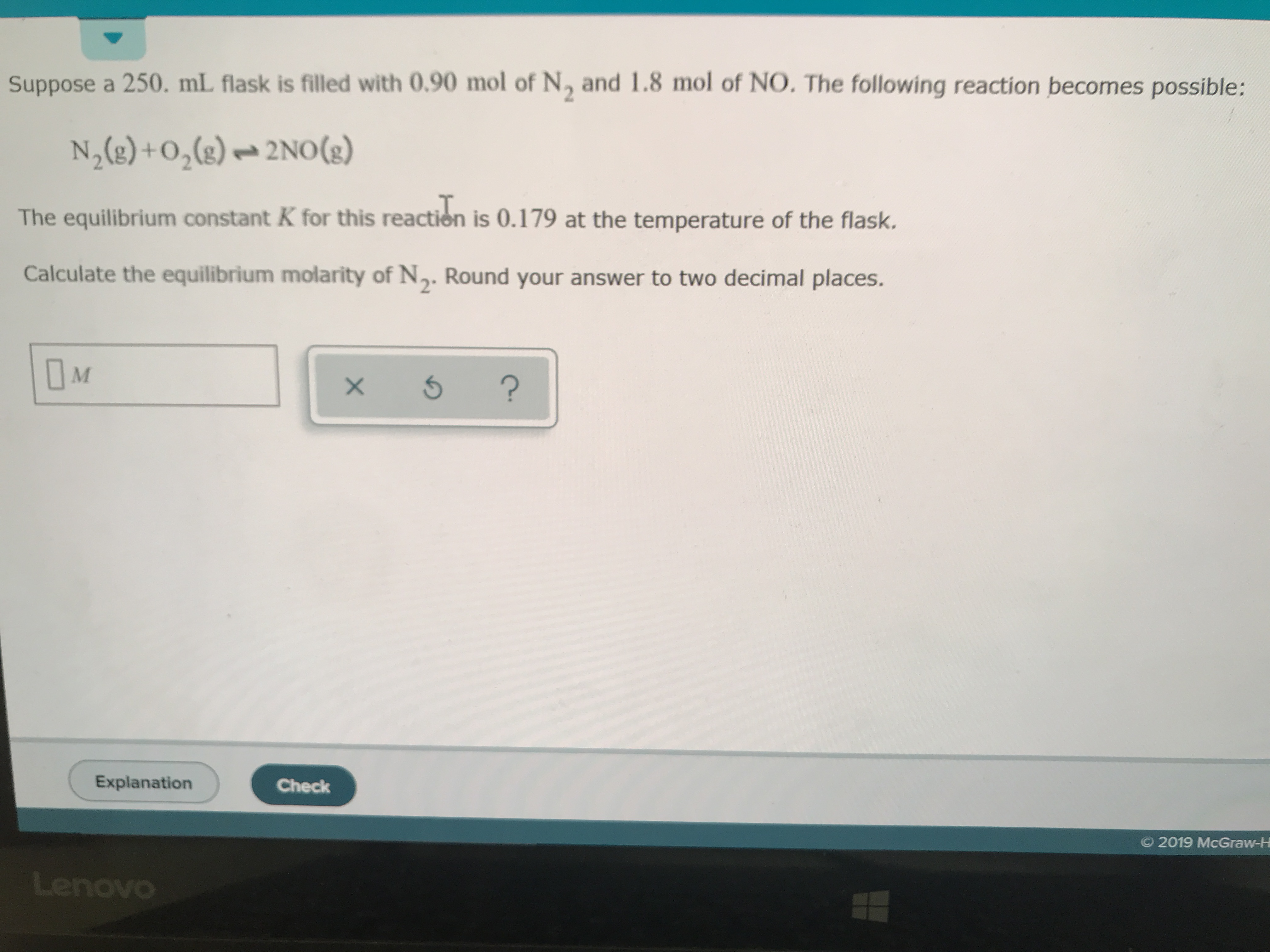# Suppose a 250 mL flask is filled with 0.90 mol N2 and 1.8 mol NO. The following reaction becomes possible: N2(g) + O2(g) <-> 2 NO(g). The equilibrium constant K for this reaction is 0.179 at the temperature of the flask. Calculate the equilibrium molarity of N2. Round your answer to two decimal places.